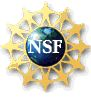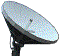Learning mathematics is like learning to swim, it requires active learning.

If you want to learn how to swim, you must get into the water.

You cannot learn to swim by watching others.

• Working with other students in small groups is strongly recommended.
• In this course, one must actively work many problems, and practice, practice, practice!

Click here to obtain information on the Math Assistance Center.

Click here to obtain the MATH M118 Mentoring Schedule.

Click here to obtain information about the Learning Center and Mentoring opportunities.

Chapter Topics

 CHAPTER TOPIC Chapter 1.1 Propositions and Truth Tables Chapter 1.2 Conditional Statements and Arguments Chapter 1.3 Chapter Review Chapter 2.1 Sets and Subsets Chapter 2.2 Set Operations Chapter 2.3 Venn Diagrams Chapter 2.4 Chapter Review Chapter 3.1 Trees and Equally Likely Outcomes Chapter 3.2 Permutations Chapter 3.3 Combinations Chapter 3.4 Chapter Review Chapter 4.1 Basic Concepts Chapter 4.2 Conditional Probability and Independence Chapter 4.3 Bayes' Theorem Chapter 4.4 Bernoulli Trials Chapter 4.5 Chapter Review Chapter 5.1 Central Tendency Chapter 5.2 Expected Value and Standard Deviation Chapter 5.3 Normal Random Variable Chapter 5.4 Normal Approx to a Binomial Random Variable Chapter 5.5 Chapter Review Chapter 6.1 System of Linear Equations in Two Variables Chapter 6.2 Matrices and Systems of Linear Equations Chapter 6.3 Matrix Notation, Algebra, and Inverses Chapter 6.5 Chapter Review Chapter 9.1 Transition Matrices Chapter 9.2 Regular Markov Chains

This site is made possible by funding from the National Science Foundation (DUE-9981111).